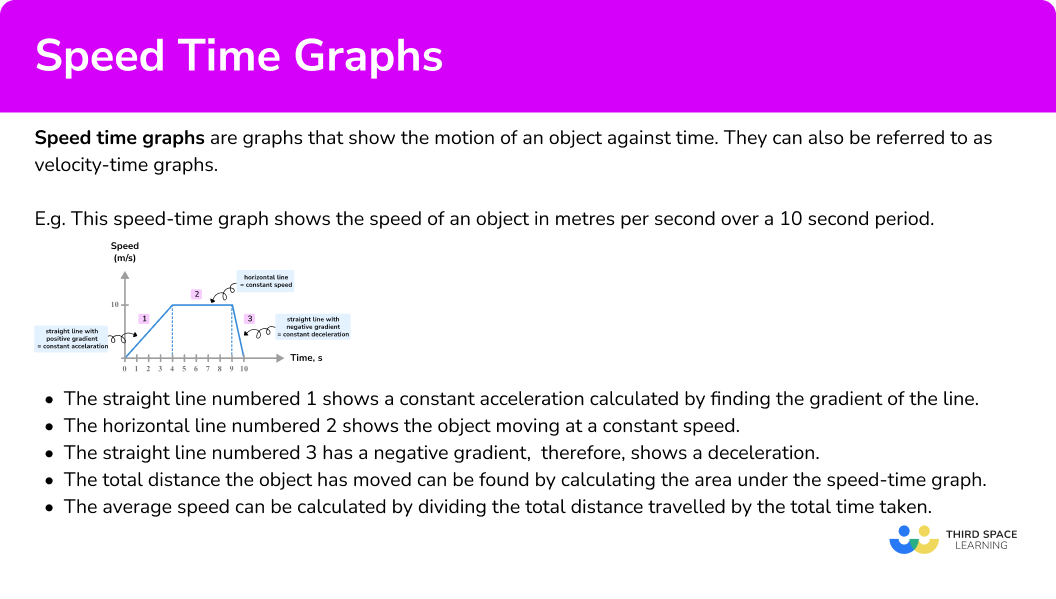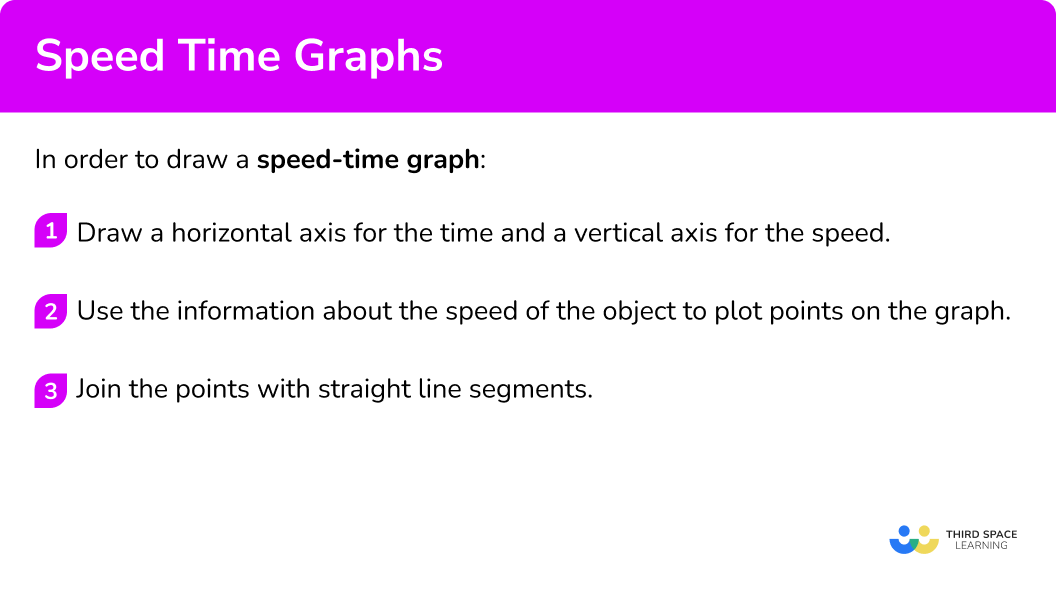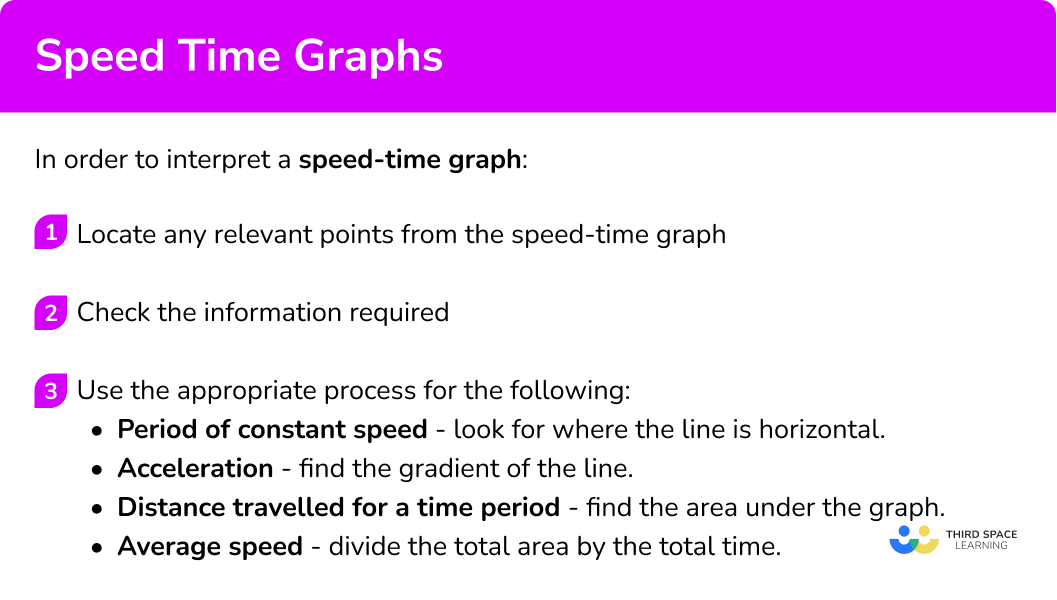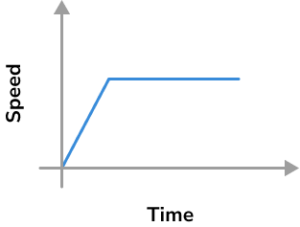# Speed Time Graph

Here we will learn about a speed-time graph including drawing, and reading from speed-time graphs. We will also learn about finding acceleration and distance travelled from a speed-time graph.

There are also speed-time graph worksheets based on Edexcel, AQA and OCR exam questions, along with further guidance on where to go next if you’re still stuck.

## What is a speed time graph?

A speed time graph is a graph that shows the motion of an object against time.

They can also be referred to as velocity-time graphs.

The units of speed used for a speed-time graph can vary, but the most common are kilometres per hour (km/h), metres per second (m/s) and miles per hour (mph).

E.g.

This speed-time graph shows the speed of an object in metres per second over a 10 second period.

The object accelerated from rest at a constant rate for 4 seconds until it reached a speed of 10 \ m/s. It then remained travelling at 10 \ m/s for a further 5 seconds before then decelerating to rest in 1 second. (Decelerating is when an object slows down.)

The straight line numbered \bf{1} shows a constant acceleration. This acceleration can be calculated by finding the gradient of the line.

Acceleration is the rate of change in speed over time. Here is the formula

\text{Acceleration }= \frac{\text{change in speed}}{\text{change in time}}

The horizontal line numbered \bf{2} shows the object moving at a constant speed.

The straight line numbered \bf{3} has a negative gradient, therefore, shows a deceleration (the object is slowing down).

The total distance the object has moved can be found by calculating the area under the speed-time graph.

The average speed can be calculated by dividing the total distance travelled by the total time taken.

### What is a speed time graph?## How to draw a speed time graph

In order to draw a speed time graph:

1. Draw a horizontal axis for the time and a vertical axis for the speed.
2. Use the information about the speed of the object to plot points on the graph.
3. Join the points with straight line segments.

### Explain how to draw a speed time graph## Related lessons on rates of change

Speed time graph is part of our series of lessons to support revision on rates of change. You may find it helpful to start with the main rate of change lesson for a summary of what to expect, or use the step by step guides below for further detail on individual topics. Other lessons in this series include:

## Speed time graph examples

### Example 1: completing a speed-time graph

The speed-time graph shows the speed of a car for the first 10 seconds of a journey.

After the first 10 seconds the car maintains its speed for 20 seconds. It then decelerates at a constant rate and stops after a further 15 seconds.

Complete the speed-time graph for the journey of the car.

1. Draw a horizontal axis for the time and a vertical axis for the speed.

The graph already has axes drawn and labelled so go straight to step 2. The first part of the journey has been drawn. We can see that the car travelled for 10 seconds, starting from rest and accelerating to a speed of 12 \ m/s.

2Use the information about the speed of the object to plot points on the graph.

The car maintains the speed for 20 seconds so we need to plot a point at the same vertical height, 12 \ m/s , when the time is 30 seconds. We then must plot a point at 45 seconds with a speed of 0 \ m/s \ (10+20+15=45).

3Join the points with straight line segments.t.

### Example 2: drawing a speed-time graph

An object accelerates from rest at a constant rate for 5 seconds and reaches a speed of 8 \ m/s. The object maintains this speed for a further 6 seconds.

Draw a speed-time graph for the motion of the object.

The object reaches a maximum speed of 8 \ m/s and the total time the object has travelled is 11 seconds.

The object starts from rest, reaches 8 \ m/s at 5 seconds and remains at 8 \ m/s until 11 seconds

Plot points at (0,0), \ (5,8) and (11,8).

### Example 3: drawing a speed-time graph

At 12:00, a train is travelling at 60 \ km/h. It maintains this speed for 15 minutes and then begins to decelerate at a constant rate for 5 minutes until it reaches a speed of 30 \ km/h. It then maintains this speed for a further 20 minutes.

Draw a speed-time graph for the motion of the train.

The horizontal axis needs to start at 12:00 and end at 12:40.

The vertical axis needs to have a maximum speed of 60 \ km/h.

Plot points at (12:00,60), \ (12:15,60), \ (12:20,30) and (12:40,30).

## How to interpret a speed time graph

In order to interpret a speed time graph:

1. Locate any relevant points from the speed-time graph.
2. Check the information required.
3. Use the appropriate process for the following.

Period of constant speed – look for where the line is horizontal.

Acceleration – find the gradient of the line.

Distance travelled for a time period – find the area under the graph.

Average speed – divide the total area by the total time.

### Explain how to interpret a speed time graph## Interpreting speed time graph examples

### Example 4: finding the acceleration from a speed-time graph

The speed-time graph shows the speed of an object over a 16 second period.

Find the acceleration of the object.

The object accelerates from rest to a speed of 12 \ m/s after 16 seconds

The straight line is from (0,0) to (16,12).

The acceleration of the object is required.

Acceleration – find the gradient of the line.

The acceleration is the gradient of the line.

The gradient can be found using

\text{acceleration }= \frac{\text{change in speed}}{\text{change in time}} = \frac{12}{16}=0.75 \ m/s^2

### Example 5: finding a deceleration from a speed-time graph

The speed-time graph shows the speed of a vehicle.

Find the deceleration of the vehicle in the last part of its journey.

The vehicle decelerates between (1.5,60) and (3,0)

The deceleration is required, so this is an acceleration question.

Acceleration – find the gradient of the line.

The acceleration is the gradient of the line.

The gradient can be found using

\text{acceleration }= \frac{\text{change in speed}}{\text{change in time}}=\frac{-60}{1.5}=-40 \ km/h^2

The acceleration is negative because the vehicle is decelerating.

Therefore, the deceleration is 40 \ km/h^{2}.

### Example 6: finding the total distance and average speed from a speed-time graph

The speed-time graph shows the motion of an object.

Find the total distance travelled and the average speed of the object over its entire journey.

The speed-time graph is formed of points (0,0), \ (3,10), \ (7,10) and (9,0)

The total distance and average speed is required.

Distance travelled for a time period – find the area under the graph.

Average speed – divide the total area by the total time.

The total distance can be found by finding the area under the graph.

The shape of the graph is a trapezium, so the trapezium area formula could be used or the shape could be separated into a rectangle and two triangles.

The total distance travelled is 65 \ m.

The average speed of the object can be found using

\text{average speed }= \frac{\text{total distance}}{\text{total time}}=\frac{65}{9}=7.22 \ m/s \ (2 dp)

### Common misconceptions

• The distance travelled is just the speed multiplied by the time

A common error when finding the distance travelled is to just multiply the speed the object is travelling by the time taken. This only works if the object has been moving at a constant speed for that whole period of time.

It will not give the distance travelled if the object has been accelerating during that time. It is important to find the area under the graph to find the distance.

• The time scale

Time is always on the horizontal axis, but care needs to be taken with the scale used. Remember there are 60 seconds in one minute and 60 minutes in one hour. This means that the scale can be subdivided in many ways.

### Practice speed time graph questions

1. What does a line with zero gradient represent on a speed-time graph?

An object acceleratingAn object moving with constant speedAn object deceleratingAn object at instantaneous restIf the gradient of the line on a speed-time graph is zero, there will be zero change of speed over time, therefore the object will be moving at a constant speed.

2. To find the total distance travelled by an object using a speed-time graph you must

Multiply the maximum speed by the total timeFind the gradient of the lineFind the area under the speed-time graphMultiply the maximum speed by the time taken to reach itThe area under the speed-time graph gives the total distance travelled.

3. Which of the speed-time graphs shows an object accelerating from rest and then maintaining a constant speed.The line must start from the origin and have a positive gradient, then change to a line with zero gradient.

4. Find the acceleration from the speed-time graph.10 \ m/s^21 \ m/s^20.5 \ m/s^22 \ m/s^2\text{acceleration }= \frac{\text{change in speed}}{\text{change in time}} = \frac{10}{5}=2 \ m/s^{2}.

5. Use the speed-time graph to find the acceleration of the object in the final part of its journey.80 \ km/h^2-80 \ km/h^2-40 \ km/h^240 \ km/h^2\text{acceleration }= \frac{\text{change in speed}}{\text{change in time}} = \frac{-120}{1.5}=-80 \ km/h^{2}.

6. Find the total distance travelled from the speed-time graph.240 \ km360 \ km150 \ km120 \ kmThe shape of the graph is a trapezium, so the trapezium area formula could be used or the shape could be separated into a rectangle and two triangles.

\text{Area}=\frac{1}{2}\times (1+3) \times 120=240

### Speed time graph GCSE questions

1. The speed-time graph shows the first part of a journey by a car.(a) Write down the speed of the car at 3 seconds.

(b) Find the acceleration of the car for the first part of its journey.

After the first 6 seconds of its journey, the car maintains its speed for a further 8 seconds before then slowing down at a constant rate of 5 \ m/s^{2} until stationary.

(c) Complete the speed-time graph for the car.

(d) Find the total distance travelled by the car.

(7 marks)(a) 9 \ m/s

(1)

(b)

15 \div 6 =

(1)

2.5 \ m/s^{2}

(1)

(c)

Horizontal line drawn from (6,15) to (14,15)

(1)

Line drawn from (14,15) to (17,0)

(1)

(d)

Attempt to use trapezium area or break into separate triangles and rectangle

(1)

Distance of 187.5 \ m found

(1)

2. The speed-time graph shows the speed of a train between 12:00 and 13:30.(a) Find the total distance travelled by the train in that time.

(b) Find the average speed of the train between 12:00 and 13:30.

(5 marks)

(a)

Attempt to break shape into trapeziums, triangles, rectangles etc

(1)

Distance between 12:00 and 12:30 found as 27.5 \ km

(1)

Total distance of 67.5 \ km

(1)

(b)

(1)

45 \ km/h

(1)

3. The speed-time graph shows the speed of an object over a period of T seconds.

If the total distance travelled by the object is 154 \ metres. Find the value of T.(3 marks)

Equation using areas of triangles and rectangle or trapezium attempted

(1)

\frac{6+T}{2} \times 14=154   or  \frac{14\times3}{2} +6\times 14+ \frac{14 \times (T-9)}{2} = 154

(1)

T= 16

(1)

## Learning checklist

You have now learned how to:

• Interpret the gradient of a straight line graph as a rate of change
• Calculate gradients of graphs and areas under graphs, and interpret results in cases such as speed-time graphs (velocity-time graphs)

## Still stuck?

Prepare your KS4 students for maths GCSEs success with Third Space Learning. Weekly online one to one GCSE maths revision lessons delivered by expert maths tutors.

Find out more about our GCSE maths tuition programme.